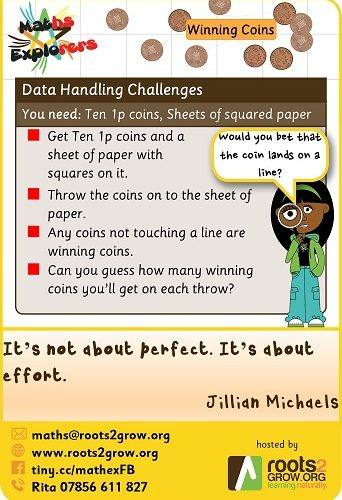# Coins in the Squares

This activity involves guessing whether coins will fall entirely within squares in a grid of squares.

Basically the idea is to throw 10 coins onto a sheet of paper. When you do this, some will probably fall off the paper. You don’t count these.

The ones that fall on the paper are the ones we are interested in. Count how many of them there are and then count how many of them fall inside the squares (i.e. not touching the grid lines).

How likely is it that coins will fall in the squares? Would you expect more than half of the coins to do this or less than half?

If this was a game at the summer fair, would you bet on a coin falling in a square or across 2 or more squares.

### The Grids

There are two grids provided here. One has grid lines that are a distance of two 1p coins apart. You can download that here – double spaced grid lines

The other one is a triple spaced grid where the distance between lines is the same as the width of 3 coins. You can download that here – triple spaced grid lines

### The Monte Carlo Method

This is a way to tell how likely it is for 1 coin to land within a square. This challenge is based on game that was played in 18th century France where people would bet on whether a coin tossed on to a tiled floor would land entirely within a tile.

This inspired a method for estimating the value of pi by throwing tooth picks onto a grid of squares. Below is a link to details about that experiment and the maths behind it for those interested.

https://www.exploratorium.edu/snacks/pi-toss

These experiments broadly fall into a category called The Monty Carlo Method. The Monte Carlo Method is a way of sampling random events to estimate answers to difficult questions. The above method of estimating pi is an example of the Monte Carlo method. Below is a computer based way to do a similar estimation.

So if you were asked to bet on how many coins out of ten would land completely within a square on double grid lined paper, what number would you choose?

What about if it were on triple grid lined paper?

Try out this activity and let us know how you got on. You can do this in one of 2 ways:

1. Add a comment to this post
2. Tweet using #momentofmaths